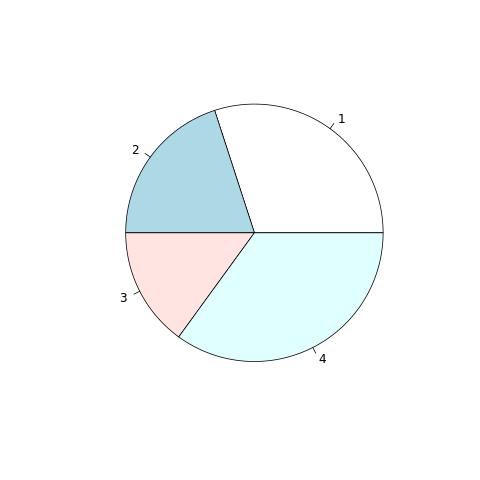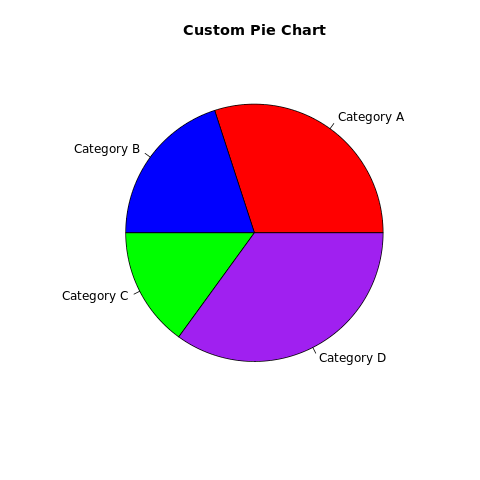# Pie Chart in R

A pie chart is a circular graph that represents data as slices of a pie. Each slice's size corresponds to the proportion of data it represents within the whole dataset.

Pie charts are often used to show the composition of a whole in terms of percentages or proportions. The proportions are represented by slices of a pie, and the size of each slice is proportional to the value of the category.

In R, pie charts can be created using the pie() function. The syntax is as follows:

pie(x)
1. x is the name of the variable that contains the categories.

For example, the following code creates a pie chart of the number of students in each grade:

This will create a pie chart with the grade variable plotted on the y-axis. The slices of the pie will represent the number of students in each grade.

## pie() function

The pie() function has many options that can be used to customize the appearance of the pie chart. These options can be used to change the colors of the slices, the labels on the slices, and the direction of the pie.

For example, the following code changes the colors of the slices to red, yellow, and green:

pie(grade, col = c("red", "yellow", "green"))
1. The col option specifies the colors of the slices.

In R, you can create pie charts using the base graphics system or various packages, such as ggplot2 and plotly.

## Create a Pie Chart in R using Base Graphics

To create a pie chart using the base graphics system, you can use the pie() function.

# Example: Creating a pie chart using base graphics data <- c(30, 20, 15, 35) pie(data)

In this example, the data vector contains the values you want to visualize as a pie chart.

## Customize the Pie Chart

You can customize the pie chart by adjusting parameters and adding labels and titles.

# Example: Customizing the pie chart using base graphics labels <- c("Category A", "Category B", "Category C", "Category D") colors <- c("red", "blue", "green", "purple") pie(data, labels = labels, col = colors, main = "Custom Pie Chart")

In this example, the labels parameter provides labels for each slice, and the col parameter sets custom colors for each slice. The main parameter sets the title.

### Full Source | R Pie chart

# Example: Creating a pie chart using base graphics data <- c(30, 20, 15, 35) # Create the pie chart pie(data) # Example: Customizing the pie chart using base graphics labels <- c("Category A", "Category B", "Category C", "Category D") colors <- c("red", "blue", "green", "purple") pie(data, labels = labels, col = colors, main = "Custom Pie Chart")

### Output:## Pie Chart in R using ggplot2

### Install and Load Required Packages

install.packages("ggplot2") library(ggplot2)

### Create a Pie Chart using ggplot2

To create a pie chart using ggplot2, you can use the geom_bar() function with a polar coordinate system.

# Example: Creating a pie chart using ggplot2 data <- data.frame(category = c("A", "B", "C", "D"), value = c(30, 20, 15, 35)) ggplot(data, aes(x = "", y = value, fill = category)) + geom_bar(stat = "identity", width = 1, position = "stack") + coord_polar(theta = "y")

### Full Source | R Piechart

# Install and load the ggplot2 package install.packages("ggplot2") library(ggplot2) # Example: Creating a pie chart using ggplot2 data <- data.frame(category = c("A", "B", "C", "D"), value = c(30, 20, 15, 35)) # Create the pie chart ggplot(data, aes(x = "", y = value, fill = category)) + geom_bar(stat = "identity", width = 1, position = "stack") + coord_polar(theta = "y")

### Output:## Create a Pie Chart in R using plotly

### Install and Load Required Packages

install.packages("plotly") library(plotly)

### Create a Pie Chart using plotly

To create an interactive pie chart using plotly, you can use the plot_ly() function.

# Example: Creating an interactive pie chart using plotly data <- data.frame(category = c("A", "B", "C", "D"), value = c(30, 20, 15, 35)) plot_ly(data, labels = ~category, values = ~value, type = "pie")

### Full Source | R Pie Chart Plotly

# Install and load the plotly package install.packages("plotly") library(plotly) # Example: Creating an interactive pie chart using plotly data <- data.frame(category = c("A", "B", "C", "D"), value = c(30, 20, 15, 35)) # Create the interactive pie chart plot_ly(data, labels = ~category, values = ~value, type = "pie")

### Conclusion

Pie charts in R can be created using the base graphics system's pie() function, the ggplot2 package with polar coordinates, or the plotly package for interactive visualizations. Pie charts are suitable for displaying proportions and percentages in a circular format. By customizing colors, adding labels, and using different packages, you can create informative and visually appealing pie charts for data analysis and presentation.